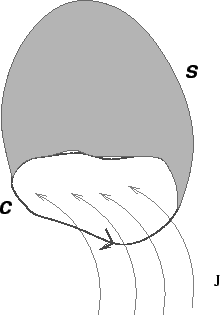Amperes Law: Biot-Savart's law for magnetic field due to a current element is difficult to visualize physically as such elements cannot be isolated from the circuit which they are part of. Andre Ampere formulated a law based on Oersted's as well as his own experimental studies. Ampere's law states that  the line integral of magnetic field around any closed path equalstimes the current which threads the surface bounded by such closed path. . Mathematically,In spite of its apparent simplicity, Ampere's law can be used to calculate magnetic field of a current distribution in cases where a lot of information exists on the behaviour of. The field must have enough symmetry in space so as to enable us to express the left hand side of (1) in a functional form. The simplest application of Ampere' s law consists of applying the law to the case of an infinitely long straight and thin wire.

Ampere's Law in Differential Form: We may express Ampere's law in a differential form by use of Stoke's theorem, according to which the line integral of a vector field is equal to the surface integral of the curl of the field,The surfaceis any surface whose boundary is the closed path of integration of the line integral.n terms of the current density, we have,whereis the total current through the surface. Thus, Ampere's lawis equivalent towhich givesYou may recall that in the case of electric field, we had shown that the divergence of the field to be given by. In the case of magnetic field there are no free sources (monopoles). As a result the divergence of the magnetic field is zeroThe integral form of above is obtained by application of the divergence theoremThus the flux of the magnetic field through a closed surface is zero.